Main page Department of Mathematics and Statistics of University of Helsinki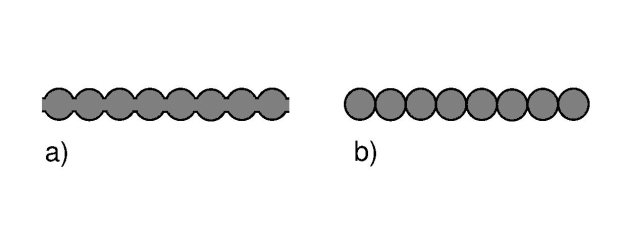Figure 1. Waveguides.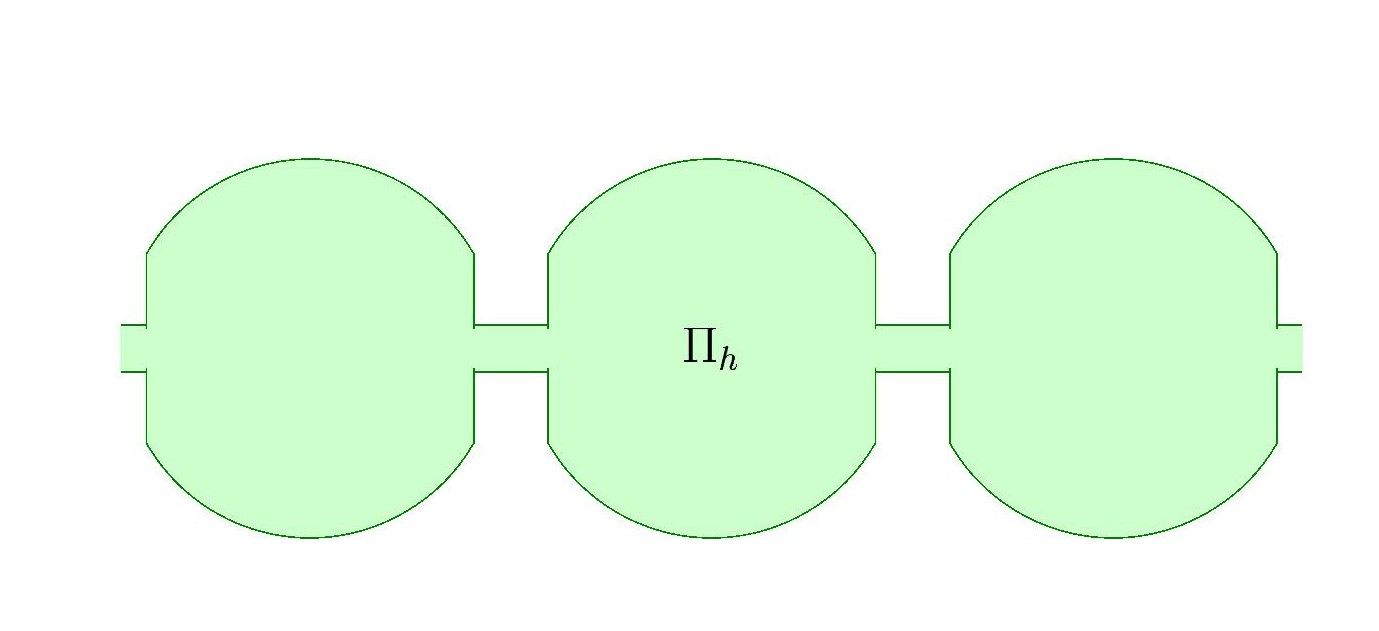Figure 2. Waveguides.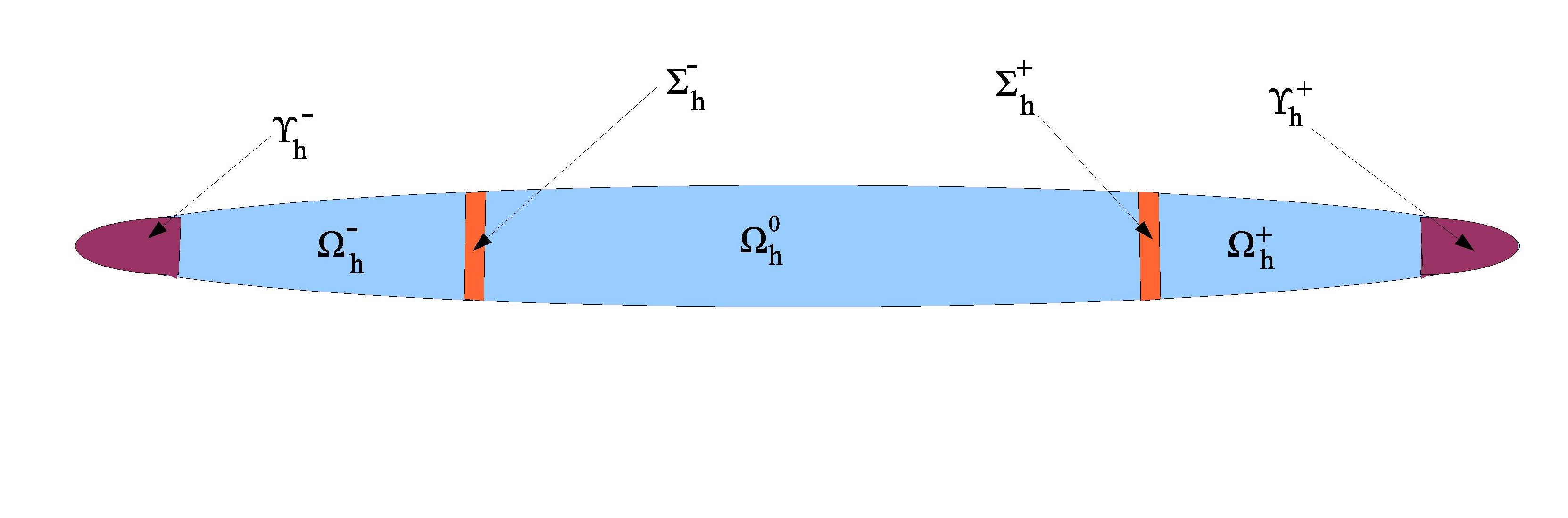Figure 3. Thin rod with rounded edges.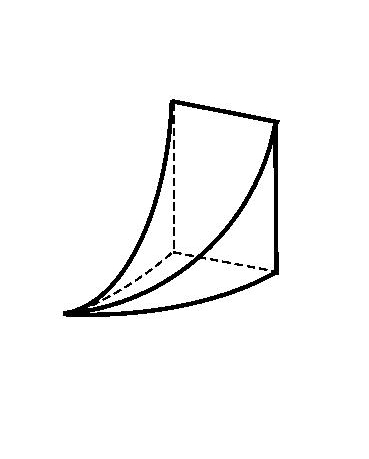Figure 4. Domain of beak shape.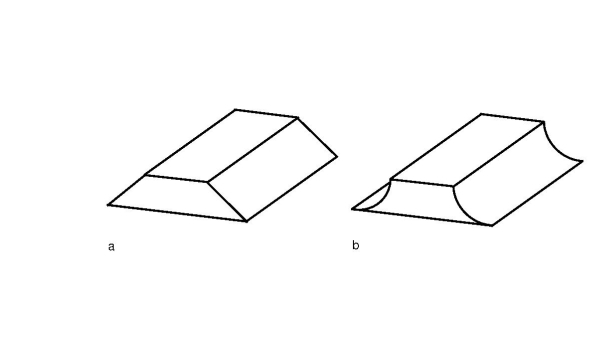Figure 5. Corner and cuspidal domains. We study the linear spectral elasticity and piezoelectricity problems. In the case of unbounded domains (waveguides) the main results concern gaps in the essential spectra. Other results include existence of essential spectrum in the case of some cuspidal bounded domains. SOME RECENT ARTICLES G.Leugering, S.A.Nazarov, A.S.Slutskij, J.Taskinen: Asymptotic analysis of a bit brace shaped junction of thin rods. To appear in Z. Angew. Math. Mech ZAMM We present a 1-D model of a junction of five thin elastic rods forming the shape of a bit brace (hand drill), or, a crankshaft. The distinguishing feature of this junction is the existence of the so called movable elements, which are rods and knots requiring modifications of the classical asymptotic ansatze. These consist of constant longitudinal displacements on the edges of the skeleton of the junction and affect the transmission conditions at its nodes. We provide asymptotic formulas for the displacements, stresses and elastic energy, as well as error estimates. An exact solution of the model is given for a particular loading. Preprint. G.Leugering, S.A.Nazarov, J.Taskinen: Umov-Poynting-Mandelstam radiation conditions in periodic composite piezoelectric waveguides. Asymptotic Anal. 111, 2 (2019), 69-111. We develop and investigate radiation conditions at infinity for the linear piezoelectricity problem in unbounded periodic domains. The approach is based on the Mandelstam radiation principle according to which the energy flux at infinity is directed away from the source. We discuss the special cases, where the more conventional radiation conditions fail, and provide examples containing purely elastic insulating and conducting inclusions as well as special cases of purely elastic and acoustic waveguides. Here is a preprint. F.L.Bakharev, J.Taskinen: Bands in the spectrum of a periodic elastic waveguide. Zeitschrift Angew.Math.Phys. 68 (2017). We study the spectral linear elasticity problem in an unbounded periodic waveguide, which consists of a sequence of identical bounded cells connected by thin ligaments of diameter of order h >0. The essential spectrum of the problem is known to have band-gap structure. We derive asymptotic formulas for the position of the spectral bands and gaps, as h approaches 0, so the method is different from some of our other papers, where max-min-principle is applied; for example the Applicable Analysis-paper, 2010. In both cases, approximate eigenfunctions are constructed, but in connection with the max-min-principle, they are formed by more straightforward ways by using cut-off functions related to the geometry of the domain, whereas in the present paper the asymptotic representation is by far more involved and also leads to more accurate results. In particular we have a sufficient condition which guarantees that a spectral band does not degenerate into an eigenvalue of infinite multiplicity. Here is a preprint. S.A.Nazarov, J.Taskinen: Elastic and piezoelectric waveguides may have infinite number of gaps in their spectra. Comptes Rendus Mécanique. 344 (2016), 190--194. We consider elastic and piezoelectric waveguides composed from identical beads threaded periodically along a spoke converging at infinity. We show that the essential spectrum constitutes a non-negative monotone unbounded sequence and thus has infinitely many spectral gaps. Here is a preprint. S.A.Nazarov, J.Taskinen: Spectral gaps for periodic piezoelectric waveguides. Zeitschrift Angew.Math.Phys. 66 (2015), 3017-3047 We construct a family of periodic piezoelectric waveguides depending on a small geometric parameter h > 0 , with the following property: as h -> 0 , the number of gaps in the essential spectrum of the piezoelectricity problem grows unboundedly. The method is similar to that in the Applicable Analysis 2010-paper, but there is a major technical complication caused by the a priori non-seldadjointness of the piezoelectricty problem. We use a self-adjoint reduction scheme developed earlier by S.Nazarov. However, this leads to a new non-local term in the reduced elliptic self ajoint boundary problem. This complicates the use of the max-min-principle, but it turns out possible to derive the needed estimates by using suitable Hardy-type inequalities and proper, special weighted Sobolev norms, among other things. Here is a preprint. S.A.Nazarov, A.S.Slutskij, J.Taskinen: Korn inequality for a thin rod with rounded ends. Math.Methods Appl.Sci. 37, 16 (2014), 2463-2483 We consider an elastic rod (Fig.3) with rounded ends and diameter proportional to a small parameter h >0 The roundness of the ends is described by an exponent 0 < m < 1 . We derive for the rod an asymptotically sharp Korn inequality with a special weighted anisotropic norm. Weight factors with m-dependent powers of h appear both in the anisotropic norm and the Korn inequality itself, and we discover three critical values m = 1/4, m=1/2 and m=3/4 at which these exponents are crucially changed. S.A.Nazarov, K.Ruotsalainen, J.Taskinen: Essential spectrum of a periodic elastic waveguide may contain arbitrarily many gaps Applicable Anal. 89,1 (2010), 2019-124. In this work the domain Ω ⊂ R³ is an unbounded periodic waveguide (see Fig.1.b), like a necklace, which consists of an infinite "needle" and an infinite number of bounded "beads" (translates of a fixed bounded cell). We make the geometry of the domain to depend on a positive parameter h > 0, which describes the distance of two adjacent beads. Due to the unboundedness of the domain, the essential spectrum S of the elasticity problem is not empty. Using general results one can show that the spectrum S has a band-gap structure: it is the union of infinitely many (bounded) intervals, or bands, I(k) ⊂ R⁺. Our aim becomes to show that the intervals I(k) do not overlap, implying the existence of spectral gaps. To this end, on the limit h → 0, the spectral elasticity problem corresponds to the case where the domain is bounded and Lipschitz, and the spectrum is discrete. Considering the cases with h > 0 as a perturbation of the limit case, we are able to control the lower and upper bounds of spectral bands I(k) and assure that for small enough h, spectral gaps are created in a controlled way. G.Cardone, S.A.Nazarov, J.Taskinen: Criteria for the existence of the essential spectrum for beak-shaped elastic bodies. J.Math. Pur. Appl. 92, 6 (2009), 628-650. An example of a beak shaped domain Ω in R³ is presented in Fig.4. The boundary irregularity is angular in two (horizontal) dimensions and cuspidal in one (vertical) dimension. The geometric parameter γ > 0 describes the cuspidal sharpness; if it were equal to 0, the beak would be turned into a corner domain. We consider the spectral problem for the linear elasticity system in the domain Ω with traction-free boundary conditions, see Formula 1. The main result of the paper, Theorem 4.2 claims that the essential spectrum is nonempty, if and only if γ ≥ 1. For 0 < γ < 1 the spectrum is thus fully discrete. The main technical device is a new weighted anisotropic Korn-type inequality. We end the paper by a discussion of the possibility of cuspidal structures to act as "black holes" for elastic waves. Our results give a mathematical explanation to the following phenomenon: hitting a trapezoidal metal brick with a hammer, the brick does cling, but a similar brick with cuspidal edges does not (see Fig.5). G.Cardone, S.A.Nazarov, J.Taskinen: `Absorption'' effect for elastic waves by the beak-shaped boundary irregularity. Doklady Physics 54, 3 (2009), 146-150. This is related to the previous paper. Back to main page .